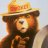# MVTI Indicator for ThinkorSwim#### J007RMC

##### Well-known member
2019 Donor
VIP
I kept the upper portion to this study it does a great job of coloring candles based on candle strength.Code:
``````#MVTI indicator BY :Kevin Morgan,,,  ARTICLE SYNOPSIS ...Many technical indicators can be used to detect trends. Active traders need information about a trend on multiple time-frame charts at a glance. Here's a bar color-coded visual trend indicator that combines the use of ADX and CCI and gives you instant multi-timeframe, high-resolution trend state information so you can invoke "with the trend" trad...
#thinkScript code from A Visual Indicator by Kevin D. Morgan, May 2019thinkScript 1: #Directional

# 20:57 0FrankB3: Hacked the MVTI indicator:: https://tos.mx/sgWjzi
#6/28/2019

input CCILength = 21;
input CCILimit = 100;
def DIPlus = reference DIPlus(length = ADXLength);
def DIMinus = reference DIMinus(length = ADXLength);
if Tr == 1 and DIPlus > DIMinus then 1 else
if Tr <= 0 and DIPlus > DIMinus and ADX > ADX then 1 else
if Tr == -1 and DIMinus > DIPlus then -1 else
if Tr >= 0 and DIMinus > DIPlus and ADX > ADX then -1
else 0;
def CCI = CCI(21, CCILimit, CCILimit).CCI;
def OverBought = CCILimit;
def OverSold = -CCILimit;
def OB = if (CCI > OverBought) then 1 else 0;
def OS = if (CCI < OverSold) then 1 else 0;
DefineGlobalColor("StrongUp", Color.GREEN);
DefineGlobalColor("Up", Color.LIME);
DefineGlobalColor("Neutral", Color.WHITE);
DefineGlobalColor("Down", Color.DOWNTICK);
DefineGlobalColor("StrongDown", Color.RED);
AssignPriceColor(
if (Tr == 1 and OB == 1) then
GlobalColor("StrongUp")
else if Tr == 1 then
GlobalColor("Up")
else if Tr == -1 and OS == 0 then
GlobalColor("Down")
else if (Tr == -1 and OS == 1) then
GlobalColor("StrongDown")
else
GlobalColor("Neutral"));``````

Last edited by a moderator:
•markosMidpoint Moving Average Indicator Questions 2